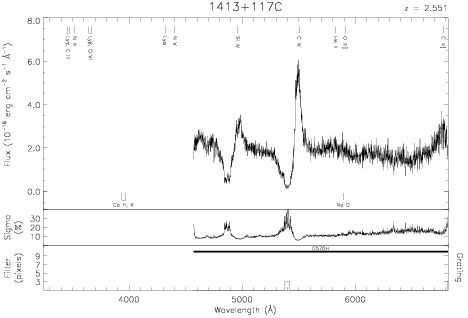Download dataset
TELESCOP= 'HST     '
INSTRUME= 'FOS     '
EQUINOX =               2000.0
DATE-OBS= '1993-06-27T14:45:32'
RA_PNT  =       213.9427800417
DEC_PNT =        11.4956430556
PA_PNT  =       218.4218000000
OBJECT  = '1413+117C'
ROOTNM01= 'Y1FB0306T'
GRNDMD01= 'SPECTROSCOPY'
DETECT01= 'AMBER   '
APERID01= 'B-1     '
FGWAID01= 'H57     '
ROOTNM02= 'Y1FB0307T'
GRNDMD02= 'SPECTROSCOPY'
DETECT02= 'AMBER   '
APERID02= 'B-1     '
FGWAID02= 'H57     '
ROOTNM03= 'Y1FB0308T'
GRNDMD03= 'SPECTROSCOPY'
DETECT03= 'AMBER   '
APERID03= 'B-1     '
FGWAID03= 'H57     '
ROOTNM04= 'Y1FB030AT'
GRNDMD04= 'SPECTROSCOPY'
DETECT04= 'AMBER   '
APERID04= 'B-1     '
FGWAID04= 'H57     '